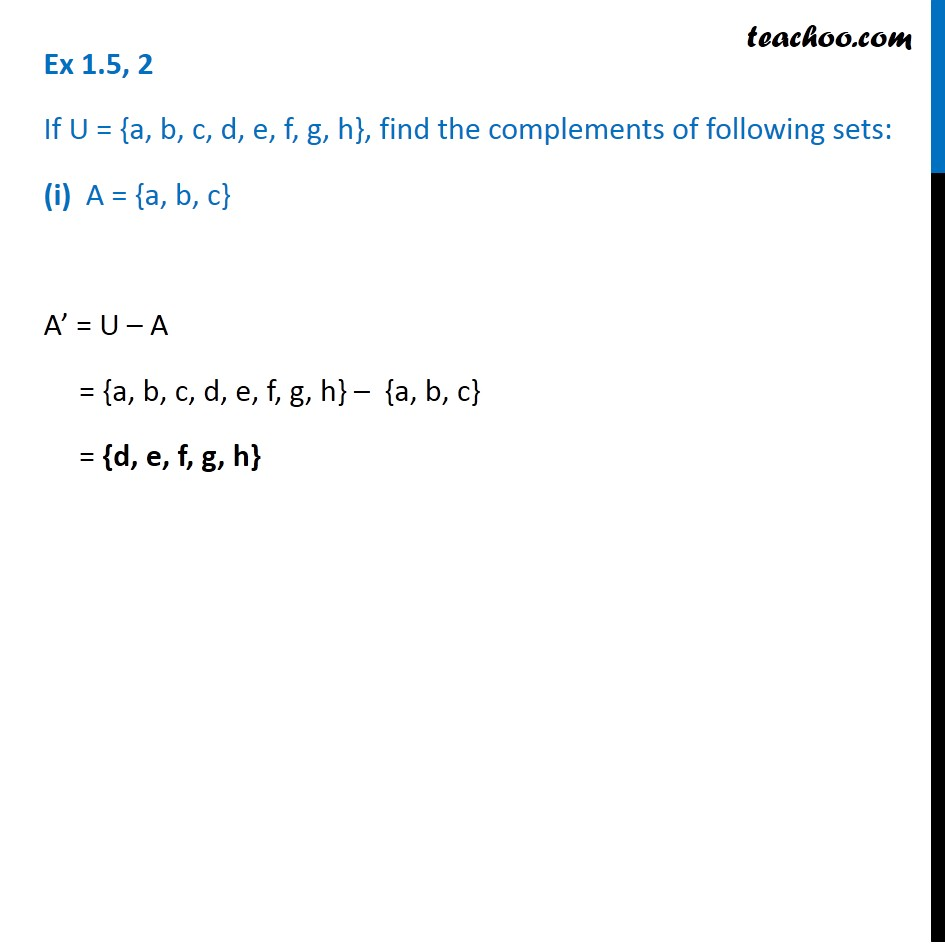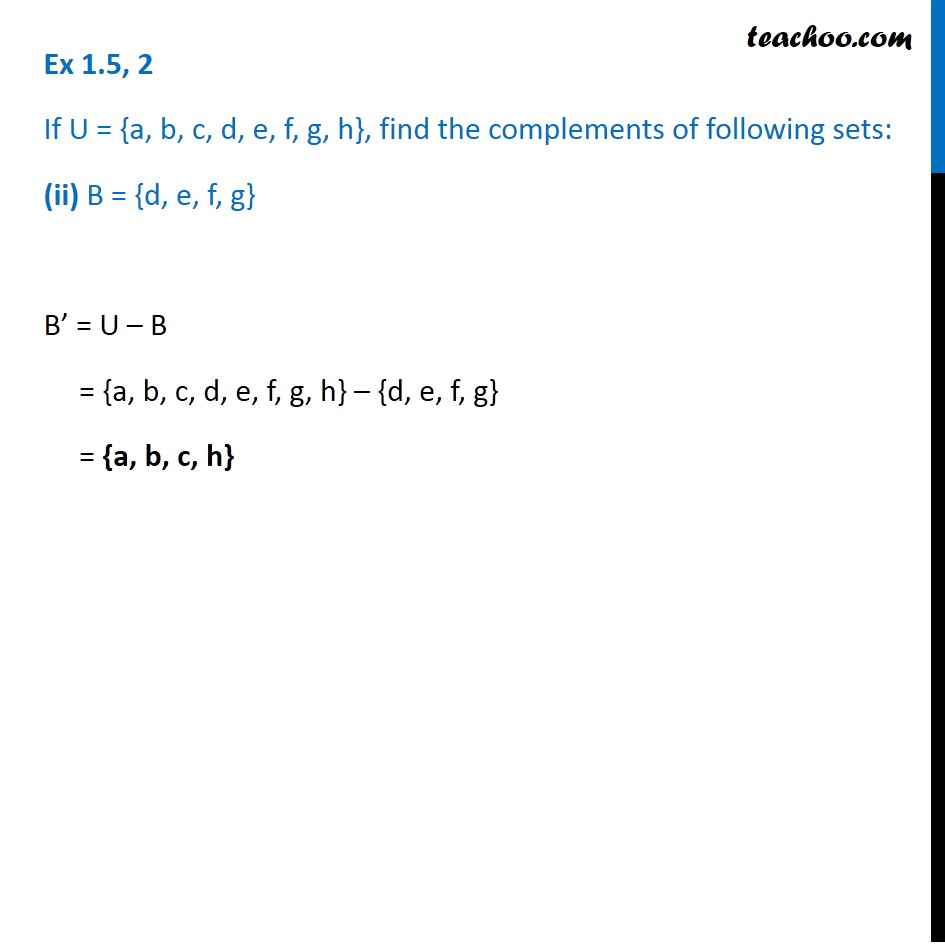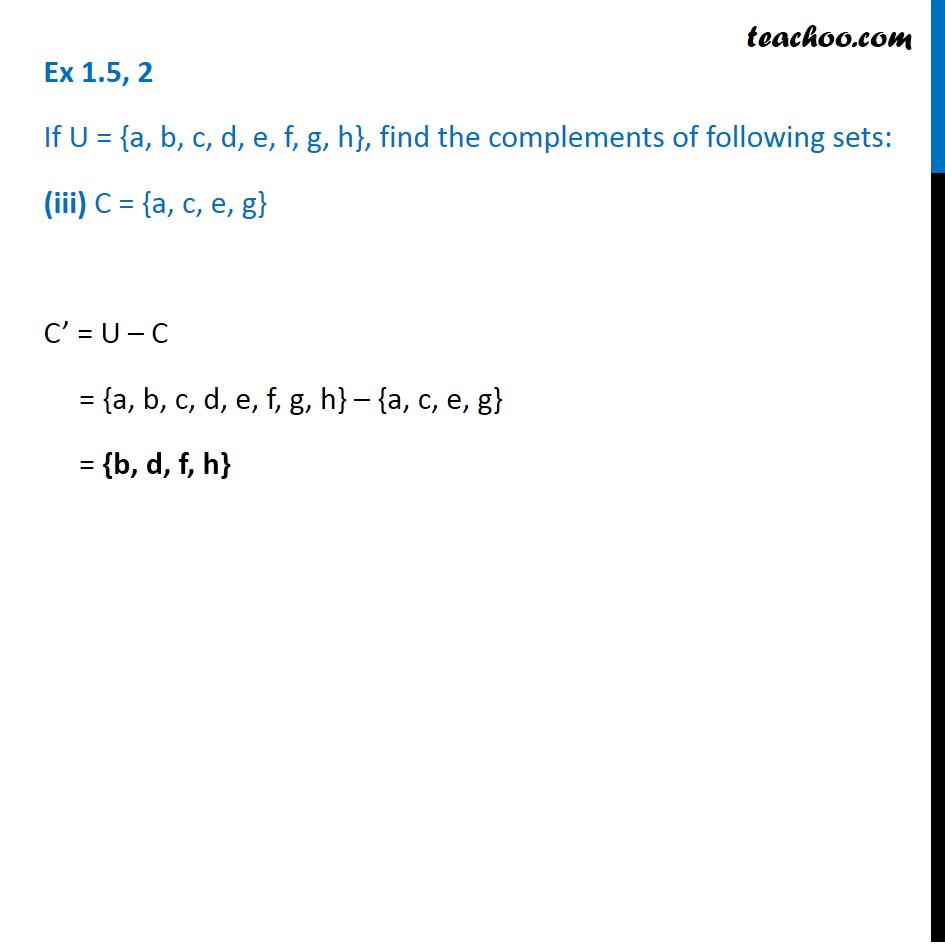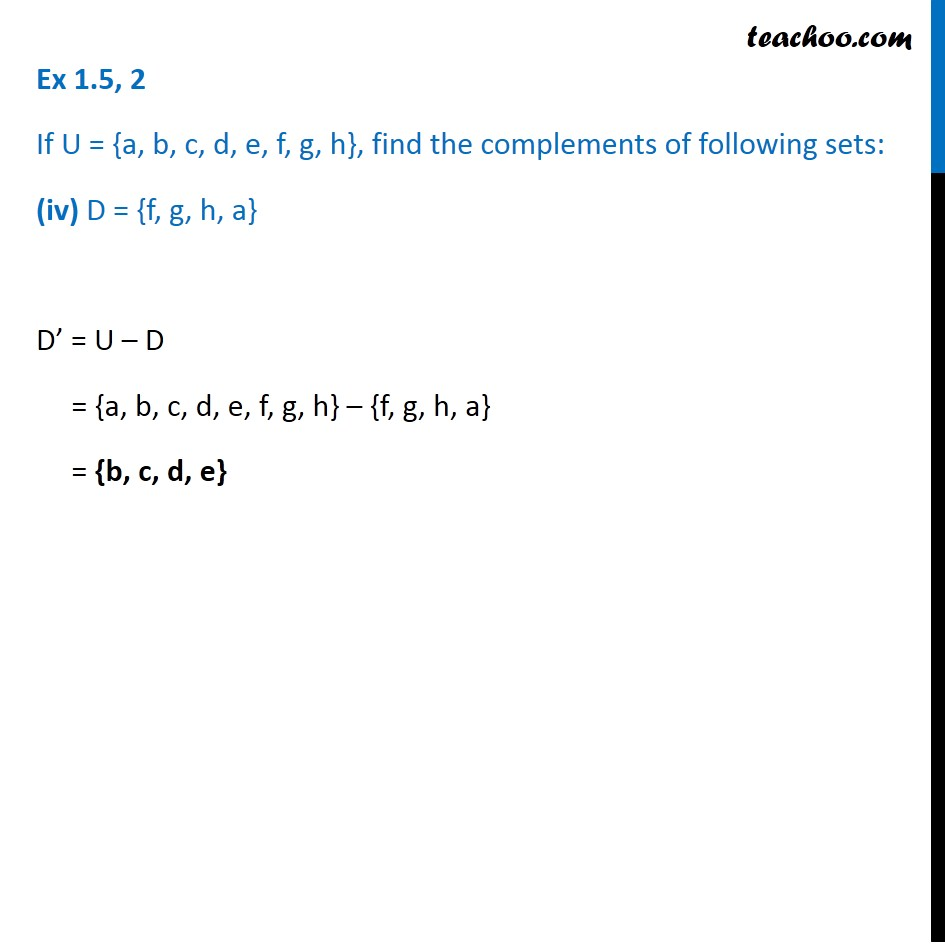Ex 1.5

Chapter 1 Class 11 Sets (Term 1)
Serial order wise641 students have Teachoo Black. What are you waiting for?

### Transcript

Ex 1.5, 2 If U = {a, b, c, d, e, f, g, h}, find the complements of following sets: (i) A = {a, b, c} A’ = U – A = {a, b, c, d, e, f, g, h} – {a, b, c} = {d, e, f, g, h} Ex 1.5, 2 If U = {a, b, c, d, e, f, g, h}, find the complements of following sets: (ii) B = {d, e, f, g} B’ = U – B = {a, b, c, d, e, f, g, h} – {d, e, f, g} = {a, b, c, h} Ex 1.5, 2 If U = {a, b, c, d, e, f, g, h}, find the complements of following sets: (iii) C = {a, c, e, g} C’ = U – C = {a, b, c, d, e, f, g, h} – {a, c, e, g} = {b, d, f, h} Ex 1.5, 2 If U = {a, b, c, d, e, f, g, h}, find the complements of following sets: (iv) D = {f, g, h, a} D’ = U – D = {a, b, c, d, e, f, g, h} – {f, g, h, a} = {b, c, d, e}